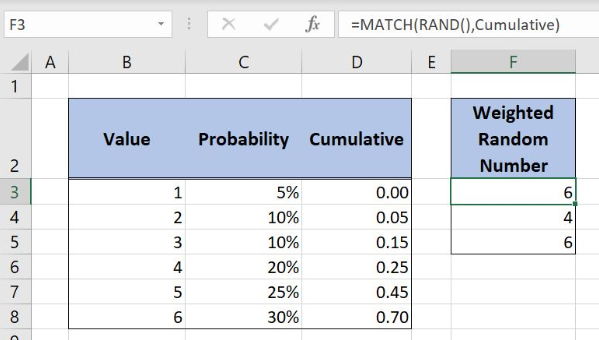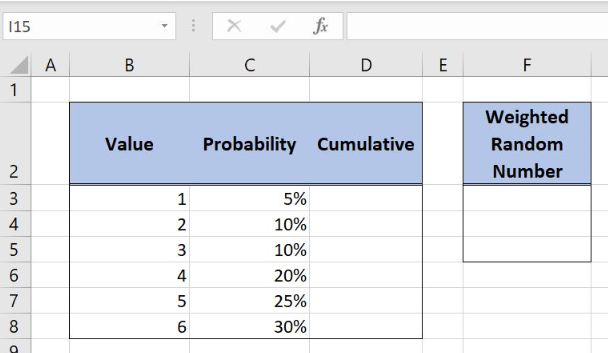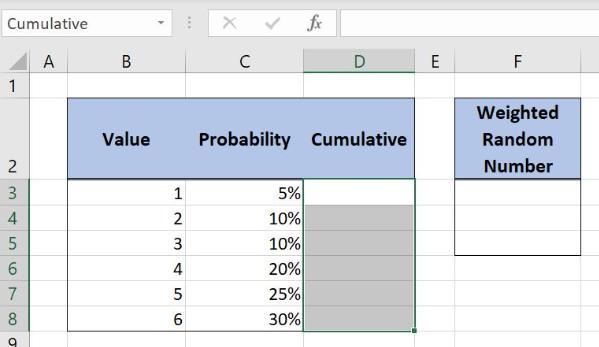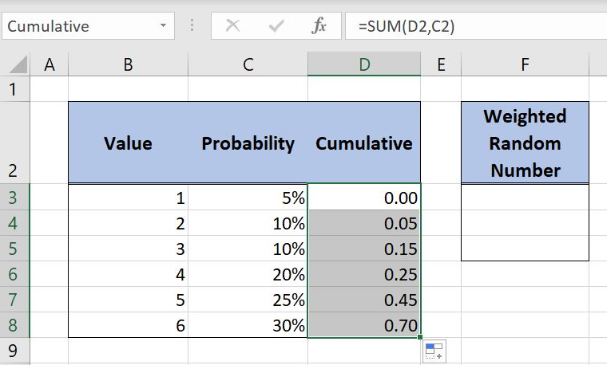Get instant live expert help with Excel or Google Sheets“My Excelchat expert helped me in less than 20 minutes, saving me what would have been 5 hours of work!”

#### Post your problem and you’ll get expert help in seconds.

Your message must be at least 40 characters
Our professional experts are available now. Your privacy is guaranteed.

# Learn How to Create a Weighted Random Number Generator in Excel

Excel allows us to create a weighted random number generator using the SUM, MATCH and RAND functions. This step by step tutorial will assist all levels of Excel users to get the random weighted numbers from the list and to place them in the separate column.Figure 1. The final result of the formula

## Syntax of the SUM Formula

`=SUM(number1, [number2], ...)`

The parameters of the SUM function are:

• number1, [number2] – numbers to sum

## Syntax of the MATCH Formula

`=MATCH(lookup_value, lookup_array, [match_type])`

The parameters of the MATCH function are:

• lookup_value – a value which we want to find in the lookup_array
• lookup_array – the array where we want to find a value
• [match_type] – a type of match. We put 0 which is an exact match.

## Syntax of the RAND Formula

The generic formula for the RAND function is:

`=RAND()`

The function returns a random decimal number between 0 and 1 and has no parameters.

## Setting up Our Data to Get a Weighted Random Number

Our table consists of 3 columns: “Value” (column B), “Probability” (column C) and “Cumulative” (column D). In column “Cumulative” we will place the cumulative probability starting from the value 0. The second table has column “Weighted Random Number” (column F) where we will extract the weighted random numbers from column B.Figure 2. Data for the example

## Get Weighted Random Number with SUM, MATCH and RAND Functions

We want to get the random weighted values from column B and to place the results in the column F. In order to make the formula more clear, we will create a named range Cumulative for cell range D3:D8.

To create a named range we should follow the steps:

• Select the cell range that should be named
• Click on the name box in Excel
• Write the name for the cell range and press enterFigure 3. Creating a named range Cumulative for column “Cumulative”

The formula in Column D looks like:

`=SUM(D2,C2)`

The SUM function calculates the cumulative probability starting from number 0. This is important for MATCH formula part and will be explained later. Also, values in column D should be sorted out in the ascending order.

To apply the formula, we need to follow these steps:

• Select cell D3 and click on it
• Insert the formula: `=SUM(D2,C2)`
• Press enter
• Drag the formula down to the other cells in the column by clicking and dragging the little “+” icon at the bottom-right of the cell.Figure 4. Using the SUM formula to get a cumulative probability starting from 0

Now we can place the formula in the cell F3 to get the random weighted number:

`=MATCH(RAND(),Cumulative)`

The parameter lookup_value in the MATCH function is a formula RAND() while the lookup_array is the named range Cumulative. The match_type is omitted because we want the approximate match.

To apply the formula, we need to follow these steps:

• Select cell F3 and click on it
• Insert the formula: `=MATCH(RAND(),Cumulative)`
• Press enter
• Drag the formula down to the other cells in the column by clicking and dragging the little “+” icon at the bottom-right of the cell.Figure 5. Extract random weighted number with SUM, MATCH and RAND functions

The RAND function returns the random number between the 0 and 1. The MATCH function searches RAND function result in the column “Cumulative”. As we can see, argument match_type is omitted and MATCH function is searching for an approximate match in the column D. That’s why the cumulative probability is starting from the value 0.

In order to cover all numbers between 0 and 1 (RAND function results) value in the cell D3 must start from 0. The formula output is number 6 because the RAND function result is somewhere between the value 0.70 and 1.

Most of the time, the problem you will need to solve will be more complex than a simple application of a formula or function. If you want to save hours of research and frustration, try our live Excelchat service! Our Excel Experts are available 24/7 to answer any Excel question you may have. We guarantee a connection within 30 seconds and a customized solution within 20 minutes.

Solution examplesI need a formula that will check if 2 or more cells containing names match then add the adjacent cells to matching name together.
Solved by F. D. in 59 minsI need help with a vlookup type formula that will take a given name and reference it against a list of names in columns D-O. then take a given date and reference it against dates in columns A10-A440, then return the cell or its contents at the intersection of those that column and row.
Solved by I. H. in 13 minsI need a formula to pop at the upper left corner of a spreadsheet. If I enter the month "January," I want the column number sum of January =SUM(AB11:AB75) from another section on the same excel page to pop right below the "January" cell, and not display the formula expression, but see the \$100.
Solved by T. Q. in 40 minsCan't add (SUM) in imported numbers from bank account
Solved by F. C. in 40 minsI need a formula to combine D2 to D100 to add together a column of numbers, then take away the same amount on the same row when column E is filled. i.e. column D is a price of an item, so the formula must calculate the total, then when the item is sold an 'a' is marked next to the item in column E, the formula then must deduct this amount from the total
Solved by X. W. in 20 mins